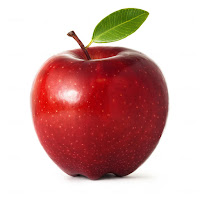## Pages

### 1.0 Physical Quantities and Units

Physics is an experimental science. And experimental science involves observing, measuring and drawing conclusion.

Lets us understand these notion. Everything that our sense organs senses are subject to observation, be it sight, smell, touch, sound or taste. When we look at an object, lets say an apple what do we observe?There are various observation that can be made given an apple,

1. There is some notion of size,
2. There is some notion of distinct colour,
3. There is some notion of quantity of matter,
4. There is some notion of smell, taste, and some sense of time when holding it for quite a while,
5. There is some notion of surface of an apple being smooth, or what about temperature.

When we look at other physical objects in our surrounding, It turns out that these are the same observational characteristic that can be asked of for other objects as well. The question then becomes if that's the case then why not might as well we introduce some general notion of measurement to make sense of an objects when we talk about these things.

Going to the definition, for any physical phenomenonal observation to which we assign a number can be called as a Physical Quantities. For eg. physical quantities that defines an apple above are weight, mass, height, temperature, surface area. It is important to remember that some physical quantities such as time, mass and so on are so fundamental that we define what it means to measure them. These are sometimes called Operational Definition.  Some of the examples are measuring a distance by a ruler, and measuring a time interval by using a stop-watch.

You might all now be thinking that, we now have a freedom a freedom to define our own scale for measurement, and indeed you are absoloutely correct but the disadvantage would be that only you would understand that scale unless you show others the operational definition. This was a huge problem in the earlier generations when people from different regions had different standard for measurement. One could only imagine having to carry his operational defintion every where he went. To resolve this difficulty people across the globe came together to standardize the measurement system.

When we measure a quantity we always have to compare it with some standard reference. Such standard reference are the unit for measurement. These units were standardized around 1960's and has since been called the International System(in French Système international d'unités) or in short SI units. The details of the SI units can be found here.

Before you move ahead i would like you to go through this, which contains tabulation of all the SI units and the derived units. Just to be clear here derived units are the units that is the combination of one or more than one base units. (Eg. of derived unit is an area, it's unit is $m^2$).

Lastly, if you have looked at all the SI unit carefully, you might find that two particular unit very puzzling. The one being unit for the amount of substance 'the mole(mol)' and the other the units for luminious intensity 'the candela(cd)'.

Definition of Mole: One mole is defined as the amount of substance containing as many elementary entities (atoms, molecules, ions, electrons, radicals, etc.) as there are atoms in 12 grams of carbon-12 (6.023 x 1023). The mass of one mole of a substance equals its relative molecular mass expressed in grams.

Definition of Candela : One candela is the luminous intensity, in a given direction, of a source that emits monochromatic radiation of frequency 540 × 1012 Hz and that has a radiant intensity in that direction of 1/683 watt per steradian.

Here are the two videos that will hopefully make your understanding on these two units much clearler.

One mole:

One Candela:

Exercise :Here are some practices exercise on calculation of moles
Here's a good notes too.

references: University Physics, Tom duncan, Nelkon and parker